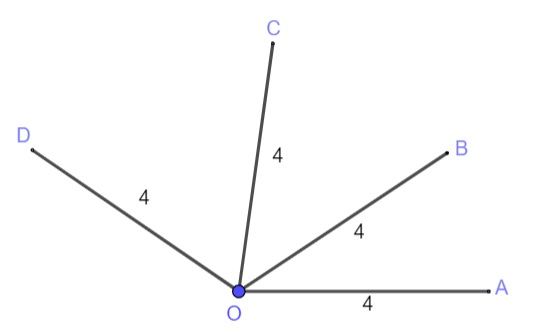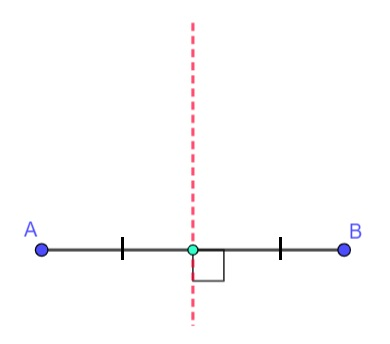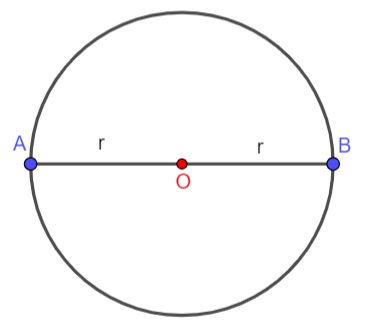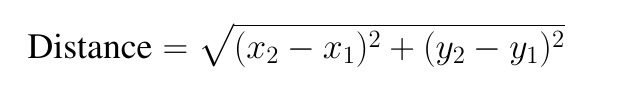Search IntMath
Close

# Equidistant Points

In geometry, two or more points are said to be equidistant from each other if they are the same distance away from a third point. The term "equidistant" comes from the Latin word "aequus," which means "equal," and "distance," which comes from the Latin word "distantia," meaning "distance."

For example, in the figure below, Points A, B, and C are all equidistant from Point D. That is because each of these points is four units away from Point D.Figure 1: Points A, B, C, and D are all equidistant from Point O.

In another example, the perpendicular bisector of any line segment is equidistant from both endpoints of the line segment.Also, the endpoints of the diameter of a circle is equidistant from the center since the lengths from the center to the circle are the radii.In order to determine if two points are equidistant from any given point, there are two approaches:

(I)You can use the distance formula to determine the lengths from the stated point to each of the points. The distance between any two points (x1,y1) and (x2,y2) is obtained using the formula:(II)You can use the midpoint formula to check if the stated point is the midpoint of the line segment joining the two endpoints.

The midpoint of a line segment with endpoints (x1,y1) and (x2,y2) is obtained using the formula:## Conclusion:

In conclusion, being able to understand what it means for points to be equidistant from each other is important in geometry and has many real-world applications. Next time you are looking at a map or trying to reason your way through a problem, remember that two points are equidistant from a third point, then the third point is halfway the distance between the two points!

## FAQ

### What is an equidistant in geometry?

In geometry, two or more points are said to be equidistant from each other if they are the same distance away from a third point.

### What is the origin of the word 'equidistant'?

The term "equidistant" comes from the Latin word "aequus," which means "equal," and "distance," which comes from the Latin word "distantia," meaning "distance.

### How do I determine if two points are equidistant?

You can check if points are equidistant by using the distance formula or the midpoint formula.

## Problem SolverThis tool combines the power of mathematical computation engine that excels at solving mathematical formulas with the power of GPT large language models to parse and generate natural language. This creates math problem solver thats more accurate than ChatGPT, more flexible than a calculator, and faster answers than a human tutor. Learn More.